##帐号 自动登录 找回密码 密码 立即注册
 搜索

# 新版Python16讲课后作业的最后一题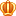发表于 3 天前 | 显示全部楼层 |阅读模式

### 马上注册，结交更多好友，享用更多功能^_^

x

import random

i = input('请输入抛硬币的次数：')
i = int(i)
print('开始抛硬币实验')

count = 1
a = 0
b = 0
c = 0
c_sub = 0
d = 0
d_sub = 0
result_sub = 0
if i <= 100:
while count <= i:
result = random.randint(1,2)
if result != result_sub:
c_sub = 1
d_sub = 1
else:
if c < c_sub:
c = c_sub
elif d < d_sub:
d = d_sub

result_sub = result
count += 1
if result == 1:
print('正面',end='')
a += 1
c_sub += 1
else:
print('反面',end='')
b += 1
d_sub += 1

else:
while count <= i:
result = random.randint(1,2)
if result != result_sub:
c_sub = 1
d_sub = 1
else:
if c < c_sub:
c = c_sub
elif d < d_sub:
d = d_sub

result_sub = result
count += 1
if result == 1:
a += 1
c_sub += 1
else:
b+= 1
d_sub += 1

print('一共模拟了',i,'次抛硬币，结果如下：')
print('正面：',a,'次')
print('反面：',b,'次')
print('最多连续正面：',c,'次')
print('最多连续反面：',d,'次')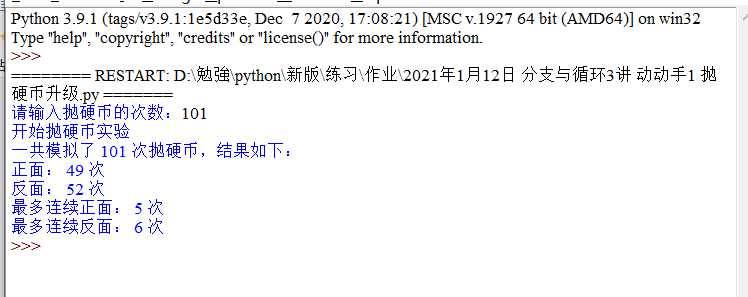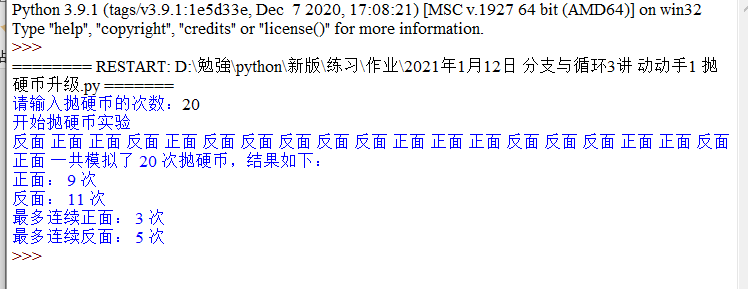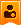楼主| 发表于 前天 00:32 | 显示全部楼层
 没有大佬么=。=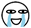发表于 前天 01:01 | 显示全部楼层
 没仔细看你的代码，但是这道题的意思就是获取两个不同的值进行判断正面或者反面。 比如小甲鱼的1，10取2的余数，只会存在 0 和 1 这两种可能 当然你也可以用0， 100 取值，0-49 为正面，50 - 100 为反面 你自己的方法也是可以的，无非就是模拟硬币的双面投掷。并没觉得有什么实质性的差距，每一种方式，只要你能保证两面的可能性都为 50% 就可以了发表于 前天 08:27 | 显示全部楼层
 ''' 楼主写的应该是没问题的，但是感觉比较乱 我自己刚刚敲了一个 楼主可以看看是不是有帮助 给楼主个建议 还是少用abcd这种变量 记不住谁是谁 ''' import random num = int(input('请输入抛硬币的次数：')) print('开始抛硬币实验') #将数据用列表存储 # up 和 1 表示正面 # down 和 2 表示反面 # [1,0,0]分别表示[正面,正面次数,正面最大连续次数] list_up = [1,0,0] list_down = [2,0,0] #循环内临时变量，用于判断 max_up = max_down = 0 for i in range(num):     random_num = random.randint(1,2)         if random_num == list_up:         list_up += 1         max_up += 1         max_down = 0         #如果随机数为1则正面次数加一         #正面连续次数加一         #反面连续次数清零         if max_up >=list_up:             list_up = max_up             #如果正面连续次数大于等于已记录列表内最大连续次数             #则更正列表内最大连续次数     #以下相同        elif random_num == list_down:         list_down += 1         max_down += 1         max_up = 0         if max_down >=list_down:             list_down = max_down              print('一共模拟了',num,'次抛硬币，结果如下：') print('正面：',list_up,'次') print('反面：',list_down,'次') print('最多连续正面：',list_up,'次') print('最多连续反面：',list_down,'次') 复制代码

 您需要登录后才可以回帖 登录 | 立即注册 本版积分规则 回帖后跳转到最后一页

GMT+8, 2021-1-17 22:44